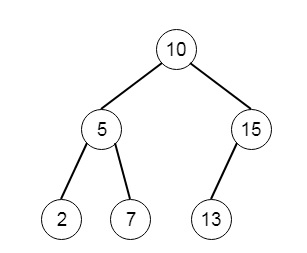# Kth Smallest Element in a BST in Python

Suppose we have a binary search tree. We have to find the Kth smallest element in that BST. So if the tree is like −So if we want to find 3rd smallest element, then k = 3, and result will be 7.

To solve this, we will follow these steps −

• create one empty list called nodes
• call solve(root, nodes)
• return k – 1th element of nodes
• the solve method is created, this takes root and nodes array, this will work as follows −
• if root is null, then return
• solve(left of root, nodes)
• add value of root into the nodes array
• solve(right of root, nodes)

Let us see the following implementation to get better understanding −

## Example

Live Demo

class TreeNode:
def __init__(self, data, left = None, right = None):
self.data = data
self.left = left
self.right = right
def insert(temp,data):
que = []
que.append(temp)
while (len(que)):
temp = que
que.pop(0)
if (not temp.left):
temp.left = TreeNode(data)
break
else:
que.append(temp.left)
if (not temp.right):
temp.right = TreeNode(data)
break
else:
que.append(temp.right)
def make_tree(elements):
Tree = TreeNode(elements)
for element in elements[1:]:
insert(Tree, element)
return Tree
class Solution(object):
def kthSmallest(self, root, k):
nodes = []
self.solve(root,nodes)
return nodes[k-1]
def solve(self, root,nodes):
if root == None:
return
self.solve(root.left,nodes)
nodes.append(root.data)
self.solve(root.right,nodes)
ob1 = Solution()
tree = make_tree([10,5,15,2,7,13])
print(ob1.kthSmallest(tree, 3))

## Input

[10,5,15,2,7,13]
3

## Output

7